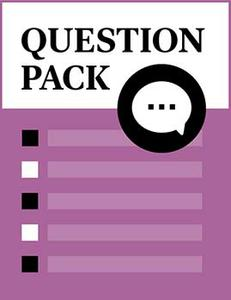# Dimension, Perspective and Projection

Source: Saylor

Student Price: Free with Top Hat Pro Subscription

This question pack asks students to apply their knowledge of computer aided design in relation to dimension, perspective and projection.

Question 23

Orthographic projections in Asia and many parts of the world EXCEPT the United States are generally placed in which quadrant?

A

B

C

D

Question 24

Orthographic projections in the United States are generally placed in which quadrant?

A

B

C

D

Question 25

A

Combining ideas

B

Refining sketches

C

Testing

D

Developing simple computer models

Question 26

Section lines are drawn at how many degrees?

A

30 degrees

B

45 degrees

C

60 degrees

D

90 degrees

Question 27

Section lines are NOT used for which of the following?

A

Thin parts

B

Thick parts

C

Cylindrical parts

D

Question 28

Section views are used to do which of the following?

A

Reveal internal details

B

Reveal external details

C

Show hidden lines

D

Show visible lines

Question 29

Stamped parts are generally shown using how many principle views?

A

One

B

Two

C

Three

D

None of the above

Question 30

The cross‑section of a feature stays normal to the path in which solid modeling process?

A

Extrusion

B

Sweeping

C

Revolving

D

Blending

Question 31

The general section line represents which of the following?

A

Iron

B

Aluminum

C

Copper

D

Zinc

Question 32

Third angle projections are associated with what types of units?

A

English units

B

International System of Units (SI)

C

Both A and B

D

None of the above

Question 33

What aspect of an engineering design does the designer typically model?

A

Function

B

Structure

C

Both A and B

D

None of the above

Question 34

Which of the following dimensions is used for heights, widths, and diameters?

A

Size dimensions

B

Location dimensions

C

Mating dimensions

D

None of the above

Question 35

Which of the following dimensions is used for parts that fit together?

A

Size dimensions

B

Location dimensions

C

Mating dimensions

D

None of the above

Question 36

Which of the following dimensions is used for placing features of components relative to each other?

A

Size dimensions

B

Location dimensions

C

Mating dimensions

D

None of the above

Question 37

Which of the following is generally the central view used in CAD?

A

Front view

B

Top view

C

Right view

D

Left view

Question 38

Which of the following is NOT a pictorial projection?

A

Perspective projection

B

Isometric projection

C

Auxiliary projection

D

Oblique projection

Question 39

Which of the following is omitted in section views?

A

Visible lines

B

Hidden lines

C

Center lines

D

Phantom Lines

Question 40

Which of the following projections is also known as Cabinet projection?

A

Perspective projections

B

Isometric projections

C

Oblique projections

D

None of the above

Question 41

Which of the following projections uses the concept of vanishing point?

A

Perspective projections

B

Isometric projections

C

Auxiliary projections

D

Oblique projections

Question 42

Which of the following projections uses the concept receding lines drawn at 30 degrees?

A

Perspective projections

B

Isometric projections

C

Auxiliary projections

D

Oblique projections

Question 43

Which of the following represents a dimension of .200 plus and minus .004?

A

Limit dimension

B

Unilateral tolerance

C

Bilateral tolerance

D

None of the above

Question 44

Which of the following represents a dimension of .200 plus .002 and minus 0?

A

Limit dimension

B

Unilateral tolerance

C

Bilateral tolerance

D

Transition dimension

Question 45

Which of the following views is used to give a better impression of 3D objects?

A

Orthographic projections

B

Pictorial views

C

Auxiliary views

D

None of the above

Question 46

Which type of axonometric pictorials has no equal angles?

A

Trimetric

B

Diametric

C

Isometric

D

Cabinet

Question 47

Which type of axonometric pictorials has three equal angles?

A

Trimetric

B

Diametric

C

Isometric

D

Cabinet

Question 48

Which type of axonometric pictorials has two equal angles?

A

Trimetric

B

Diametric

C

Isometric

D

Cabinet

Question 49

with which of the following?

A

Product design

B

Product development

C

Product manufacture

D

All of the above

Question 50

In mechanical engineering, design is the process of bringing together which of the following?

A

Engineering principles

B

Material resources

C

Creativity

D

All of the above

Question 51

CAD offers which of the following methods to represent edges and surfaces in x, y, and z axes?

A

2D

B

3D

C

Solid modeling

D

All the above

Question 52

CAD offers which of the following methods to represent solid geometry, fully defined three dimensional solid shapes, with free‑form curved faces, and material and mass properties?

A

2D

B

3D

C

Solid modeling

D

All the above

CC BY 3.0 - Saylor